# Understanding Elementary Shapes Class 6 Notes CBSE Maths Chapter 5 [Free PDF Download]

## Revision Notes for CBSE Class 6 Maths Chapter 5 - Free PDF Download

It is important to have excellent command over the Understanding Elementary Shapes Class 6 Notes as it not merely helps students to learn various important topics given in the chapter but also helps them to revise the chapter effectively before the exam. The experts at Vedantu are strongly engaged in formulating the optimum study material that meets the requirements of the students. We assure you that your doubts related to the topic Understanding Elementary Shapes would be cleared.

Register Online for NCERT Class 6 Science tuition on Vedantu.com to score more marks in CBSE board examination. Vedantu is a platform that provides free CBSE Solutions (NCERT) and other study materials for students. Maths Students who are looking for the better solutions ,they can download Class 6 Maths NCERT Solutions to help you to revise complete syllabus and score more marks in your examinations.

Do you need help with your Homework? Are you preparing for Exams?
Study without Internet (Offline)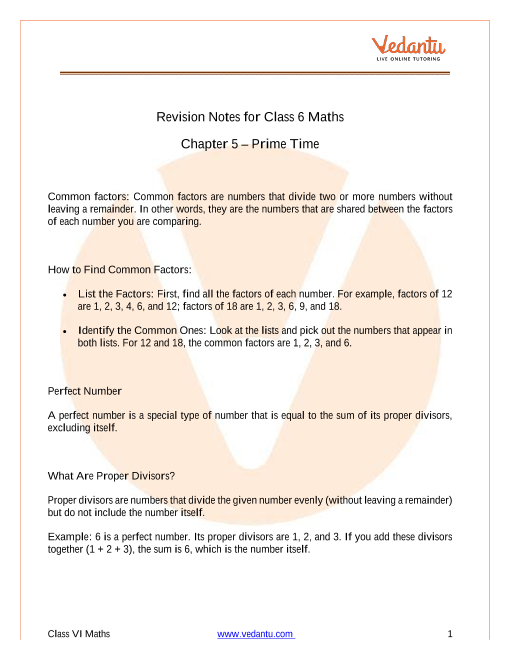## Access Class 6 Mathematics Chapter 5 - Understanding Elementary Shapes Notes in 30 Minutes

Measurement of Line Segment:

• A line segment is defined as a segment of a line that is fixed in place. This enables the measurement of a line segment.

• The length of a line segment is the distance between its endpoints.

• When comparing the lengths of line segments, a graduated ruler and a divider are used. These methods give accurate length distances.

• Also, we can compare the two line segments by observation or with the help of trace paper. But, both the methods have very little accuracy and we can’t trace the lengths every time.

Angles – ‘Right’ and ‘Straight’:

• An example of an angle is when the hand of a clock shifts from one location to another.

• A right angle is used to turn from north to east.

• A full turn is made by turning in the same direction by two straight angles (or four right angles).

• The angle for one revolution is called a complete angle.

• One revolution's angle is a full angle.

• A protractor is used to calculate the size of an angle in degrees.

• A $\dfrac{1}{4}$ revolution is a right angle, whereas a $\dfrac{1}{2}$ revolution is a straight angle.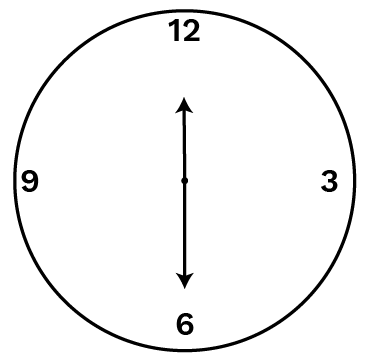(a)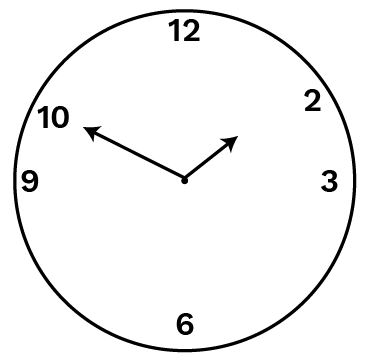(b)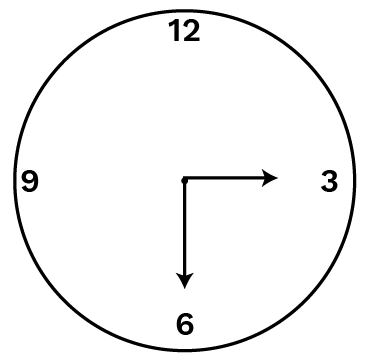(c)

• From above,

Clock $1$ represents $\dfrac{1}{2}$ of revolution or $2$ right angles.

Clock $2$ represents $\dfrac{1}{4}$ of revolution or $1$ right angles, and

Clock $3$ represents $\dfrac{3}{4}$ of revolution or $3$ right angles.

• A right angle is $\dfrac{1}{4}$ a revolution and a straight angle is $\dfrac{1}{2}$ a revolution.

• Protractor is an instrument used to measure the size of an angle in degrees.

• The measure of a right angle is ${{90}^{0}}$ and hence that of a straight angle is ${{180}^{0}}$.

• An angle is said to be acute if its measure is less than that of a right angle and is said to be obtuse if its measure is more than that of a right angle but is less than a straight angle.

• Sea-saw, rooftop, opening book, etc. are examples of an acute angle.

• House, desk for book reading, etc. are examples of an obtuse angle.

• A reflex angle is larger than a straight angle.

• Two intersecting lines are perpendicular if the angle between them is ${{90}^{0}}$.

• The perpendicular bisector of a line segment is perpendicular to the line segment that divides it into two equal parts.

Triangles Can Be Classified As Follows Based on Their Angles:

Angles – ‘Acute’, ‘Obtuse’ and ‘Reflex’:

• An acute angle is an angle smaller than a right angle.

• An obtuse angle is one that is greater than a right angle but smaller than a straight one.

• A reflex angle is more than twice as large as a straight angle.

 Nature of Angles In The Triangle Name Each angle is acute Acute angled triangle One angle is a right angle Right-angled triangle One angle is obtuse Obtuse angled triangle

Triangles can be classified as follows based on the lengths of their sides:

 Nature of Angles In The Triangle Name All three sides are of unequal length Scalene triangle Any two of the sides are of equal length Isosceles triangle All three sides are of equal length Equilateral triangle

Polygons are named based on their sides:

 Number of Sides Name of the Polygon $3$ Triangle $4$ Quadrilateral $5$ Pentagon $6$ Hexagon $8$ Octagon

Quadrilaterals are further classified with reference to their properties:

 Properties Name of the Quadrilateral One pair of parallel sides Trapezium Two pairs of parallel sides Parallelogram A parallelogram with $4$ right angles Rectangle A parallelogram with $4$ sides of equal length Rhombus A rhombus with $4$ right angles Square

Faces, Edges, and vertices:

We see around us many three-dimensional shapes. Cubes, cuboids, spheres,

Cylinders, cones, prisms, and pyramids are some of them.

• Each side of the cube is a flat surface called a flat face (or simply a face).

• Two faces meet at a line segment called an edge.

• Three edges meet at a point called a vertex.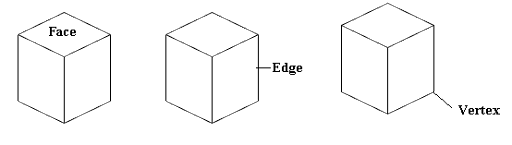Prism:

• The triangular prism has a triangular base, whereas the rectangular prism has a rectangular base.

Our experts at Vedantu had put an extensive effort to formulate Class 6 Maths Chapter 5 notes in a short and precise manner. The students can effectively use the Class 6 Maths Chapter 5 Notes to have a quick revision of the topic just before the examination.

It is mandatory to study all the important concepts related to different geometrical figures given in Understanding Elementary Shapes Class 6 Notes in order to score better marks in the exam and to have a thorough understanding of the important topics given in the chapter. As the chapter is so vast, we have segregated the chapter in different sections. A detailed explanation of every topic is provided in our Class 6 Maths Chapter 5 notes. These detailed explanations will help students solve every question given in different exercise productions.

Students can make use of Understanding Elementary Shapes notes and gain the necessary information to prepare the complete chapter in a better way. Instead of studying the last minutes, all students need to do is to refer to Class 6 Maths Chapter 5 notes, as they are very precise and all the important concepts are explained extremely well. With this, students will surely be able to answer all the Understanding Elementary Shapes questions that may come up in the examination.

Rather than cramming the last minute for their examination and getting useless stress, the Understanding Elementary Shapes notes can be used in a smart way to gain the best out of it for the exam. Students can also evaluate their strengths and weaknesses and prepare the chapter accordingly to score maximum marks in the exam. The  Understanding Elementary Shapes notes are available free of cost. Students can download  Understanding Elementary Shapes notes in a PDF format just with a  single click on the PDF link given below.

### Understanding Elementary Shapes Introduction

We can see many different shapes around us that are drawn using curves or lines. We can see open curves, close curves, edges, corners in our surroundings. We use them to form angles, circles, triangles, line segments, and polygons of different sizes and measurements. In this chapter, we will learn how geometrical shapes such as triangles, circles, polygons, etc of different sizes and measurements are constructed using a ruler or divider.

The chapter Understanding Elementary Shapes is divided into 10 sections and 9 exercises.

• The first section is the basic introduction to Understanding elementary shapes having examples from the surroundings that are formed using curves or lines.

• The second section introduces the methods of comparing two or more than two line segments of different lengths.

• The third section introduces the concept of right angle and straight angles by directions.

• The fourth section introduces different angles such as acute angle, obtuse angle, right angle, straight angle, and reflex angle.

• The fifth section discusses how to measure different angles using the protractor and ruler.

• The sixth section discusses perpendicular lines and their constructions.

• This seventh section introduces different types of a triangle like a scalene triangle, an equilateral triangle, an isosceles triangle, an acute angle triangle, an obtuse angle triangle, and a right-angled triangle.

• The eight sections introduce different types of quadrilaterals such as rectangle, square, parallelogram, rhombus, and trapezium.

• The ninth section introduces a classification of polygons on the basis of their sizes and vertices.

• The tenth section introduces three-dimensional shapes such as cone, cube, cylinder, pyramid, and sphere. This section also discusses the number of faces, edges, and vertices in different three-dimensional shapes.

### Benefits of Understanding Elementary Shapes Notes by Vedantu

• Understanding Elementary Shapes Class 6 Notes have been prepared in accordance with the latest academic year syllabus so that students don't find any confusion if any changes have been made by the CBSE board.

• Prepared by the teachers who have extensive experience and know what exactly is required to mention.

• The students will be able to observe short keynotes of the chapter that will boost their exam preparation.

• Available in a free downloadable PDF format so that students can easily refer to it when required.

• Vedantu made an effort to facilitate the students with the perfect Class 6 Maths Chapter 5 notes which definitely assure them to get better marks.

## FAQs on Understanding Elementary Shapes Class 6 Notes CBSE Maths Chapter 5 [Free PDF Download]

Q1. What do you understand about elementary shapes ?

The Chapter of Class 6 Maths which is The Elementary Shapes very important as they will be introduced to lines, angles and triangles. They will learn the types and the properties of the elementary shapes. The students will learn about a straight line which is starting from a point and will end at a fixed point. The formation of angles is by joining two line segments and the measure of angle by protractor. These are the basic geometry and the students have to learn with full attention.

Q2. What are the basic geometrical ideas?

Geometry is the study of the various shapes and their positions and measurement of the same. The basic geometrical ideas give an idea of the basic terms used. Some of them are;

• The point gives a definite location that cannot be moved.

• The line is a collection of points.

• A ray is a line that has a fixed point and can be indefinitely extended from the other direction.

• A part of a line that has a definite length is called a line segment.

• A plane is a surface of any solid that can be flat or even curved.

• Collinear and non-collinear points If three or more points lie in the same line is collinear and if three or more points do not lie in the same line are non-collinear points.

• The curve is a continuous line without any sharp edges.

• Polygons are made of the line segments which are connected.

• Angles are formed by the joining of the two rays at a point.

• A triangle is a closed plane surface with three lines segments.

Q3. What is an angle?

An angle is formed by two different rays which are joined at the point. If you are taking two rays namely AB and BC and if they are made to join at the vertex B then the angle formed can be named angle ABC. The middle letter will represent an angle. Students in Class 6 Maths will be learning different types of angles and their construction. The details of the chapter can be well captured in Vedantu from NCERT solutions. The explanations are given in easy steps and comprehensively structured.

Q4. Why should we not compare line segments by mere observation?

The two-line segments cannot be compared by mere observation.  Sometimes it might be seen as the two are of the same length but even a slight difference will make a difference in the value and the numerical might go wrong.  The mathematical calculation should be accurate and there is no guesswork. Especially when you are working on a geometrical topic you should be sure that your pencils are sharp so your value is perfect.

These solutions are available on Vedantu's official website(vedantu.com) and mobile app free of cost.

Q5. What are the properties of parallelogram and rectangle?

The main properties of a parallelogram are that;

•  Opposite sides are parallel and equal to each other, opposite angles are equal, diagonals bisect each other if one angle is 90 degrees then all the other angles will be 90.

• A parallelogram is divided by the diagonal into two congruent triangles.

• Rectangle has all angles as the right angle.

• Opposite sides are equal.

• Diagonals are equal and bisect each other.

• Every rectangle has at least one right angle and it is a parallelogram.

Students should be thorough with these properties and the differences. They should develop conceptual knowledge from the start of the chapters.SHARETWEETSHARESUBSCRIBE